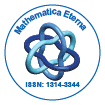Mathematica EternaOpen Access

ISSN: 1314-3344+32-466902147

Abstract

A derivation of two transformation formulas contiguous to that of Kummer’s second theorem via a differential equation approach

S. Kodavanji, A. K. Rathie, R. B. Paris

The purpose of this note is to provide an alternative proof of two transformation formulas contiguous to that of Kummer’s second transformation for the confluent hypergeometric function 1F1 using a differential equation approach.

Top